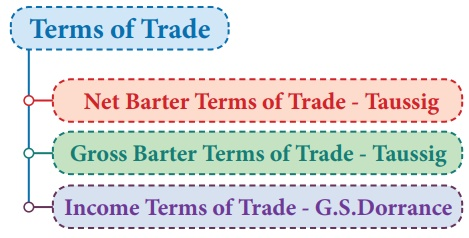Home | | Economics 12th Std | Terms of Trade

## Chapter: 12th Economics : Chapter 7 : International Economics

The gains from international trade depend upon the terms of trade which refers to the ratio of export prices to import prices.

The gains from international trade depend upon the terms of trade which refers to the ratio of export prices to import prices.## 1. Meaning

It is the rate at which the goods of one country are exchanged for goods of another country. It is expressed as the relation between export prices and import prices. Terms of trade improves when average price of exports is higher than average price of imports.

## 2. Types of Terms of Trade

The different concepts of terms of trade were classified by Gerald M.Meier into the following three categories:

Terms of Trade related to the Ratio of Exchange between Commodities### 1. Net Barter Terms of Trade

This type was developed by Taussig in 1927.The ratio between the prices of exports and of imports is called the “net barter terms of trade’. It is named by Viner as the ‘commodity terms of trade’.

It is expressed as:

Tn= (Px / Pm) x 100

Where,

Tn = Net Barter Terms of Trade

Px = Index number of export prices

Pm = Index number of import prices

This is used to measure the gain from international trade. If ‘Tn’ is greater than 100, then it is a favourable terms of trade which will mean that for a rupee of export, more of imports can be received by a country.

### 2 Gross Barter Terms of Trade

This was developed by Taussig in 1927 as an improvement over the net terms of trade. It is an index of relationship between total physical quantity of imports and the total physical quantity of exports.

Tg = (Qm/Qx) x 100

Where, Qm = Index of import quantities

Qx = Index of export quantities

If for a given quantity of export, more quantity of import can be consumed by a country, then one can say that terms of trade are favourable.

### 3 Income Terms of Trade

The income terms of trade was given by G.S.Dorrance in 1948. It is the index of the value of exports divided by the price index for imports multiplied by quantity index of experts. In other words, it is the net barter terms of trade of a country multiplied by its exports-volume index.

Ty = (Px / Pm)Qx

Where, Px = Price index of exports

Pm  = Price index of imports

Qx  = Quantity index of exports

## 3.  Terms of Trade related to the Interchange between Productive Resources

### 1 The Single Factoral Terms of Trade

Viner has devised another concept called ‘‘the single factoral terms of trade’’ as an improvement upon the commodity terms of trade. It represents the ratio of export-price index to the import-price index adjusted for changes in the productivity of a country’s factors in the production of exports. Symbolically, it can be stated as

Tf = (Px / Pm) Fx

Where, Tf stands for single factoral terms of trade index. Fx stands for productivity in exports (which is measured as the index of cost in terms of quantity of factors of production used per unit of export).

### 2 Double Factoral Terms of Trade

Viner constructed another index 1called ‘‘Double factoral terms of Trade’’. It is expressed as

Tff = (Px / Pm) (Fx / Fm)

which takes into account the productivity in country’s exports, as well as the productivity of foreign factors. Here, Fm represents import index (which is measured as the index of cost in terms of quantity of factors of production employed per unit of imports).

Tags : International Economics , 12th Economics : Chapter 7 : International Economics
Study Material, Lecturing Notes, Assignment, Reference, Wiki description explanation, brief detail
12th Economics : Chapter 7 : International Economics : Terms of Trade | International Economics